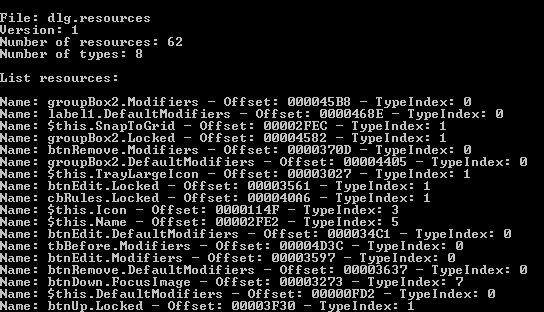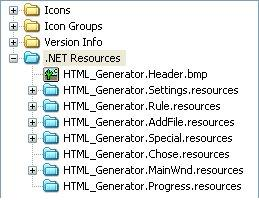.NET Manifest Resources## Introduction

Some time ago I wrote a PE Editor called CFF Explorer ('cause I needed to) with the support for .NET MetaData, since there wasn't such a tool. The only tool I could find was Asmex (which you can find on codeproject), but the problem with that tool is that you cannot modify the MetaData fields and, moreover, it relies still on the .NET Framework. And I don't say this to criticize Asmex, which is surely useful, but because I needed something different. Anyway I wrote a resource viewer for the PE Editor and wanted to show the MetaData resources as well. So, in order to do that, avoiding to use an external .NET Assembly, I had to analyze the Manifest Resource format.

Let's take a look at the Manifest Resources contained in a .NET assembly:As you can see, there can be various types of files. Reading a bitmap, for example, is very simple: every Manifest Resource begins with a dword that tells us the size of the actual embedded resource... And that's it... After that, we have our bitmap. Ok, but what about those ".resources" files? The article is all about them. In this shot there's a ".resources" file for every dialog in that .NET Assembly, this means every resource of a dialog is contained in the dialog's own ".resources" file.

Handling these compiled resources files is, using the .NET Framework, very easy. You can convert them into XML files (".resx" files) through a utility called Resgen.exe (downloadable on the MSDN) or simply use the members of the System.Resources namespace (who can also handle ".resx" files) to do whatever you want. You can create one, for example (the code is taken from the MSDN):

using System;
using System.Resources;

public class WriteResources {
public static void Main(string[] args) {

// Creates a resource writer.
IResourceWriter writer = new ResourceWriter("myResources.resources");

// Adds resources to the resource writer.

// Writes the resources to the file or stream, and closes it.
writer.Close();
}
}

Through the use of ResourceWriter it's a very easy task to create resources files. What about reading them?

using System;
using System.Resources;
using System.Collections;

public static void Main(string[] args) {

// Opens a resource reader and gets an enumerator from it.

// Goes through the enumerator, printing out the key and value pairs.
while (en.MoveNext()) {
Console.WriteLine();
Console.WriteLine("Name: {0}", en.Key);
Console.WriteLine("Value: {0}", en.Value);
}
}
}

Very easy indeed. Through the IResourceReader interface we can ask for an enumerator which gives us every resource name and value. It's also possible to load directly a specific resource from a file etc etc. So, as you can see, the Framework provides everything we need to play around with resources files. Anyway, for those of you who are still interested in knowing the internal format, go on reading.

## Resources Files Format

A very brief description. The first dword is a signature which has to be 0xBEEFCACE, otherwise the resources file has to be considered as invalid. Second dword contains the number of readers for this resources file, don't worry, it's something we don't have to talk about... Framework stuff. Third dword is the size of reader types This number is only good for us to skip the string (or strings) that follows, which is something like: "System.Resources.ResourceReader, mscorlibsSystem.Resources.RuntimeResourceSet, mscorlib, Version=1.0.5000.0, Culture=neutral, PublicKeyToken=b77a5c561934e089". It tells the framework the reader to use for this resources file.

Ok, now we got to the interesting part. The next dword tells us the version of the resources file (existing versions are 1 and 2). After the version, another dword gives the number of actual resources in the file. Another dword follows and gives the number of resource types.

To gather the additional information we need, we have to skip the resource types. For each type there's a 7bit encoded integer who gives the size of the string that follows. To decode these kind of integers you have to read every byte until you find one which hasn't the highest bit set and make some additional operations to obtain the final value... For the moment let's just stick to the format. After having skipped the types we have to align our position to an 8 byte base. Then we have a dword * NumberOfResources and each dword contains the hash of a resource. Then we have the same amount of dwords, this time with the offsets of the resource names. Another important dword follows: the Data Section Offset. We need this offset to retrieve resources offsets. After this dword we have the resources names. Well, actually it's not just the names (I just call it this way), every name  (7bit encoded integer + unicode string) is followed by a dword, an offset which you can add to the DataSection offset to retrieve the resource offset. The first thing we find, given a resource offset, is a 7bit encoded integer, which is the type index for the current resource.

## The Source Code

I put all the code in a simple class:

class CResourcesFile
{

public:

CResourcesFile();
~CResourcesFile();

UINT Size;

DWORD Version;
DWORD NumberOfResources;
DWORD NumberOfTypes;

BYTE *pTypes;
BYTE *pNamesOffsets;
BYTE *pDataSection;
BYTE *pNames;

BOOL ReadName(UINT nResource, WCHAR *Str, UINT Len);
BOOL GetResourceInfo(UINT nResource, WCHAR *Str, UINT Len,
DWORD *Offset, INT *TypeIndex);

private:

BOOL DecodeInt(BYTE *pAddress, INT *Value, UINT *uSize);
};

The use of this class is very simple, you just copy/paste the class and all its members to your project and use it someway like this:

void main()
{
TCHAR FileName[MAX_PATH];

_tprintf(_T("Resources File to open:\n"));
_tscanf(_T("%s"), FileName);

//
//

// ...

CResourcesFile ResFile;

{
return;
}

_tprintf(_T("\n\nFile: %s\n"), FileName);
_tprintf(_T("Version: %d\n"), ResFile.Version);
_tprintf(_T("Number of resources: %d\n"), ResFile.NumberOfResources);
_tprintf(_T("Number of types: %d\n"), ResFile.NumberOfTypes);

_tprintf(_T("\nList resources:\n\n"));

WCHAR ResName;

for (UINT x = 0; x < ResFile.NumberOfResources; x++)
{
DWORD Offset;
INT TypeIndex = 0;

if (ResFile.GetResourceInfo(x, ResName, 1024, &Offset, &TypeIndex))
{
_tprintf(_T("Name: %S - Offset: %08X - TypeIndex: %d\n"), ResName,
Offset, TypeIndex);
}
}

getch();
}

The first thing which has to be done is to process a resources file with the ProcessResourcesFile function:

{

//
// Collect basic information: pointer and size of the file
//

Size = uSize;

//
// Read the magic number, its value has to be: 0xBEEFCACE
//

DWORD MagicNumber;

MagicNumber = *(DWORD *) ptr;
ptr += sizeof (DWORD);

if (MagicNumber != RESOURCES_MAGIC_NUMBER)
return FALSE;

ptr += sizeof (DWORD);

ptr += sizeof (DWORD);

//
//

//

Version = *(DWORD *) ptr;
ptr += sizeof (DWORD);

//
//

NumberOfResources = *(DWORD *) ptr;
ptr += sizeof (DWORD);

//
//

NumberOfTypes = *(DWORD *) ptr;
ptr += sizeof (DWORD);

//
// Skip Types: (CHAR *Type;) * NumOfTypes
// (Save position)
//

pTypes = ptr;

for (UINT x = 0; x < NumberOfTypes; x++)
{
INT StringSize = 0;
UINT ValueSize = 0;

if (!DecodeInt(ptr, &StringSize, &ValueSize))
return FALSE;
ptr += ValueSize;

ptr += StringSize;
}

//
// Alignes position
//

DWORD Position = (DWORD) (((ULONG_PTR) ptr) - ((ULONG_PTR) pBaseAddress));

DWORD Aligned = Position & 7;

if (Aligned != 0)
{
ptr += (8 - Aligned);
}

//
// Skip name hashes
//

ptr += (sizeof (DWORD) * NumberOfResources);

//
// Skip name positions (first save location)
//

pNamesOffsets = ptr;

ptr += (sizeof (DWORD) * NumberOfResources);

//
//

DWORD DataSectionOffset;

DataSectionOffset = *(DWORD *) ptr;
ptr += sizeof (DWORD);

pDataSection = (BYTE *) (DataSectionOffset + ((ULONG_PTR) pBaseAddress));

//
// Save names position
//

pNames = ptr;

return TRUE;
}

The ReadName is just a shorter version of the GetResourceInfo, so let's just see the GetResourceInfo and skip the rest:

//
// Collect Resource Info
//

BOOL CResourcesFile::GetResourceInfo(UINT nResource, WCHAR *Str, UINT Len,
DWORD *Offset, INT *TypeIndex)
{
//
//

DWORD NameOffset = *(DWORD *) ((nResource * sizeof (DWORD)) +
((ULONG_PTR) pNamesOffsets));

if (NameOffset > (DWORD) (((ULONG_PTR) pNames) - ((ULONG_PTR) pDataSection)))
return FALSE;

ZeroMemory(Str, Len * sizeof (WCHAR));

BYTE *ptr = (BYTE *) (NameOffset + ((ULONG_PTR) pNames));

INT NameSize = 0;
UINT ValueSize = 0;

if (!DecodeInt(ptr, &NameSize, &ValueSize))
return FALSE;
ptr += ValueSize;

memcpy(Str, ptr, NameSize);

ptr += NameSize;

//
//

DWORD DataOffset = *(DWORD *) ptr;

BYTE *pData = (BYTE *) (DataOffset + ((ULONG_PTR) pDataSection));

//
// Collect info
//

if (Offset) *Offset = (DWORD) (((ULONG_PTR) pData) -

if (TypeIndex)
{
*TypeIndex = 0;
ValueSize = 0;

if (!DecodeInt(pData, TypeIndex, &ValueSize))
return FALSE;
}

return TRUE;
}

That's all, I hope it's useful.

## Post Scriptum

Of course that's not everything, if you want to handle resources, you have to threat them according to their type. This means you have to get their type from the TypeIndex. To retrieve the type string is very simple, instead of skipping all the types, you just skip the ones who come before the TypeIndex one and read the string which follows. In my CFF Explorer I support some types of resources (and display only those one) for example Bitmaps, Icons and PNGs.Have fun!

Daniel Pistelli